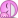# Basic Limit Results

• B
Gold Member
In my text, it states the Basic Limit Results as follows:

For any real number ##a##, and any constant ##c##,

(i) ##\lim_{x \rightarrow a}{x}=a##
(ii) ##\lim_{x \rightarrow a}{c}=c##

Now from the previous chapter, Im used to seeing these as taking the limit of some function as the x values of that function approach some x value (a). This will give some y value if a limit exists.

Now for (i), is this saying that we are taking the limit of some x value as our x values close in on some other x value (a), and the limit is the x value that we're closing in on (a)? I don't know what to make of all the x values and it seems quite confusing.

Mentor
2021 Award
The answer is yes, although I'm not sure whether it should be seen like this. I find it easiest just to consider the function ##x \longmapsto f(x)=y=x## resp. ##x \longmapsto f(x)=y=c\,.## This way you stay in the terms of functions without any "new" interpretation needed. In the first case you're approaching a point on a straight line, namely ##(x,y)=(a,a)##. In the second case you're sitting on the line ##y=c## and which ever point you approach, it will remain ##(x,y)=(*,c)##, e.g. ##(a,c)##. But you don't need this geometric view, you can as well operate with the usual definition ##\lim_{x \to a}f(x) = f(a)## and the functions I mentioned.

•opus
The answer is yes, although I'm not sure whether it should be seen like this. I find it easiest just to consider the function ##x \longmapsto f(x)=y=x## resp. ##x \longmapsto f(x)=y=c\,.## This way you stay in the terms of functions without any "new" interpretation needed. In the first case you're approaching a point on a straight line, namely ##(x,y)=(a,a)##. In the second case you're sitting on the line ##y=c## and which ever point you approach, it will remain ##(x,y)=(*,c)##, e.g. ##(a,c)##. But you don't need this geometric view, you can as well operate with the usual definition ##\lim_{x \to a}f(x) = f(a)## and the functions I mentioned.

For (i), define ##f## by ##f(x) = x## on the appropriate domain.

Then ##\lim_{x \to a} x## is shorthand for ##\lim_{x \to a} f(x)##.

For (ii), similarly, ##\lim_{x \to a} c## is shorthand for ##\lim_{x \to a} g(x)## where ##g## is given by ##g(x) = c##.

•opus
Gold Member
x⟼f(x)=y=xx \longmapsto f(x)=y=x resp. x⟼f(x)=y=c
Having a hard time deciphering this. Could you please explain?

In the first case you're approaching a point on a straight line, namely (x,y)=(a,a)(x,y)=(a,a).
Does it have to be a straight line? In drawing it out, although I'm not sure if it's accurate, it looks like this is describing the line y=x. Is this true?

In the second case you're sitting on the line y=cy=c and which ever point you approach, it will remain (x,y)=(∗,c)(x,y)=(*,c), e.g. (a,c)(a,c)

So just a horizontal line as to whatever x value we approach, it will approach the same y value?

Mentor
2021 Award
Having a hard time deciphering this. Could you please explain?
@Math_QED already did. I didn't know how you note a function:
• ##x \longmapsto \ldots \text{ expression with x } \ldots##
• ##y= \ldots \text{ expression with x } \ldots##
• ##f(x) = \ldots \text{ expression with x } \ldots##
so I combined all of them: ##x \longmapsto f(x) = y = \ldots \text{ expression with x } \ldots##
Just it have to be a straight line? In drawing it out, although I'm not sure if it's accurate, it looks like this is describing the line y=x. Is this true?
Yes. O.k. straight line is doubled, so either straight or line, but that is only a matter of language, either will do.
So just a horizontal line as to whatever x value we approach, it will approach the same y value?
Yes.

These are the graphs of the functions as stated in @Math_QED 's and my post. It is the geometry behind. If you don't want to rely on graphs, which is often a good idea esp. if the functions are weird, then you can work with the analytic definition of limits as well.

•opus
Gold Member
Sometimes these get confusing because it takes me a long time to type out my responses and it throws the whole conversation of balanceI think I got it now. Thank you guys.